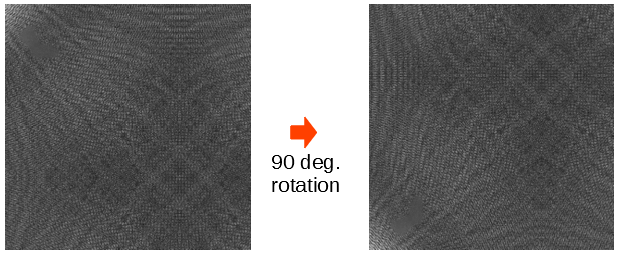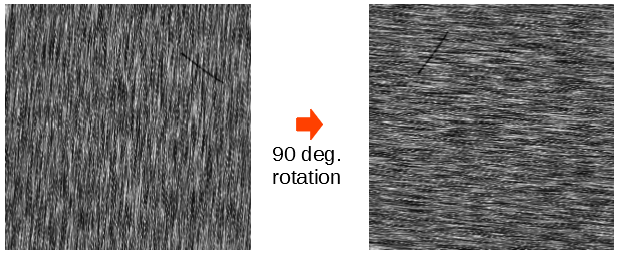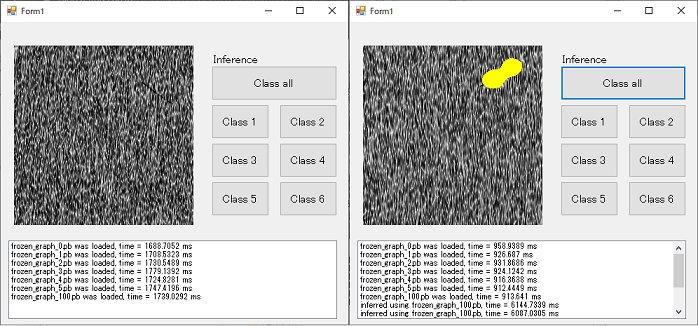# Deep Learning: Rotation invariance and multi-kind defection with different size

Hi, this is chang.

Recently, I introduced multi-kind defection using U-Net*2. After the publication, I noticed that the detection of Class_2 was bad and made an investigation. I found a bug in my source code. Bug fixing let me know some interesting characteristics of deep learning. So I made a consideration again about multi-kind defection.

0. Rotation invariance

The bug was in Viewer. Images were always rotated by 90 degree before inference.

C#/Viewer/Form1.cs(Before fixiing)

```private void btnInferAll_Click(object sender, EventArgs e)
...
arrIn[CHANNEL*(i * IMG_SIZE + j) + n] = (resized.GetPixel(i, j).R + resized.GetPixel(i, j).G + resized.GetPixel(i, j).B) / 3.0f;```

C#/Viewer/Form1.cs(After fixing)

```private void btnInferAll_Click(object sender, EventArgs e)
...
arrIn[CHANNEL*(i * IMG_SIZE + j) + n] = (resized.GetPixel(j, i).R + resized.GetPixel(j, i).G + resized.GetPixel(j, i).B) / 3.0f;```

This means other than Class_2 were successfully detected even if the images are 90 degree rotated. Here, I want to think about rotation invariance of neural network.

Translation invariance is one of the useful characteristics of convolutional neural network. It means that any location in the image is OK for defection. On the other hand, it is generally known that deep learning are not so robust against rotation and scale*3.

I guess DAGM images are created using random parameters. Their background and defects are randomly distributed. Deviation at a specific direction does not exist. So their features are never varied by image rotation. I think this is the reason that the source code including the bug (90 deg rotation) accomplished the defection with quite high level.90 deg. rotation of Class_1
Because the whole area of image is random, rotation does not change the impression.

On the other hand, many images of Class_2 have the layout like a river flow of vertical direction and cuts of horizontal direction. Rotation drastically change its impression. So the defection was failed with 90 degree rotation.90 deg. rotation of Class_2
You can see a river flow of vertical direction and cuts of horizontal direction. Rotation drastically changes its impression.

Results show that deep learning does not guarantee the rotation invariance. The bug let me learn!!! Ha ha ha..

1. Defection with different size

When a neural network learns multi-kind defection and defects have difference in size, the small defects are difficult to be learned. In case of DAGM, Class_2 and Class_3 are so. I used to normalize label with the area of defect*4. This way works well in tensorflow but not in tensorflow + keras. I guess it is becasuse the value of label is very small compared to the designated range of keras interface (-1.0 to 1.0 for image and 0.0 to 1.0 for label). So I tried the label with weight (coefficient) close to 1.0.

Class Weight
1 0.9
2 1.0
3 0.9
4 0.9
5 0.9
6 0.8

Weight for label.
Emphasize Class_2 by 1.0 and weaken Class_6 by 0.8.

In the source code, I wrote like below.

configurate_data.cpp

```vector<float> weight = {0.9, 1.0, 0.9, 0.9, 0.9, 0.8};
...
labelAll[(j*IMG_SIZE + i)*CATEGORY + n + 1] = weight[n]; // defection```

Note that I rewrite configurate_data.py in c language for speeding up.

I changed the definition of label from bool to float. Previously, I wrote that binary-crossentropy of keras only worked in boolean label. Sorry but it was misunderstanding.

```def readLabels(self, filename, DATA_SIZE):
images = np.zeros((DATA_SIZE, self.IMG_SIZE, self.IMG_SIZE, self.channels), dtype=np.float)```

I also changed the viewer a little. If you use the label of float type, output values depend on the label value. For example, the label of Class_1 is 0.9, so the outputs of its defect area are around 0.9. Therefore, it is recommended that the threshold values for defection are adjusted in proportion to weight.

viewer.cs

```private void showInferenceAll(float[] arrOut)
{
Bitmap detected = (Bitmap)resized.Clone();
for (int i = 0; i < IMG_SIZE; i++)
{
for (int j = 0; j < IMG_SIZE; j++)
{
// class 1
if (arrOut[CHANNEL * (i * IMG_SIZE + j) + 0] > 0.8) // ←this
{
detected.SetPixel(j, i, Color.Red);
}
// class 2
if (arrOut[CHANNEL * (i * IMG_SIZE + j) + 1] > 0.9) // ←this
{
detected.SetPixel(j, i, Color.Yellow);
}
```

Now Class_2 are detected.But,,,in fact, the bug fix of image rotation was all I had to do for detecting Class_2 and Class_3. I mean...Class_2 and Class_3 are detected without weighted labels. Why?............................. It is just an estimate but I think it is related to the interface of keras. Keras adjusts its outputs to the range of 0.0 to 1.0. I guess it effects like batch normalization and improve the robustness for scale variance. If the batch including small defects are normalized, it is possible to work like weighted label.

2. Afterword

Today's consideration was not clear. But it was OK to understand that float label works in binary-crossentropy of keras.

I've updated the source codes*5